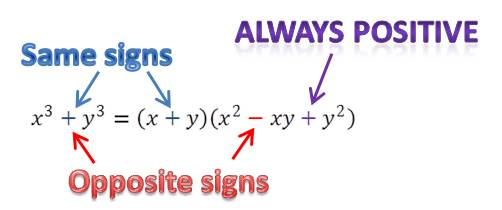## Factoring the sum or difference of two cubes

The sum or difference of two cubes can always be factored as follows:$(x^3+y^3)=(x+y)(x^2-xy+y^2)$$(x^3-y^3)=(x-y)(x^2+xy+y^2)$

These formulas are not too easy to figure out on your own, so your best approach is to memorize them. But how do you keep straight where the plus and minus signs go? Some students like to use the acronym “SOAP”, which stands for Same-Opposite-Always Positive. This means that the first sign is the same as the sign in the sum or difference of the cubes, the second sign is the opposite of this sign, and the third sign is always positive.Algebra 2
Blue Taste Theme created by Jabox